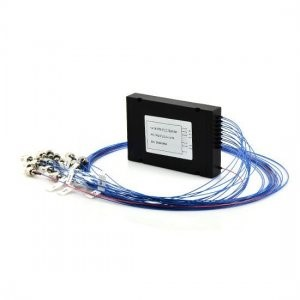# 武汉申玛-保偏光纤分路器

• 来源:SunmaFiber.COM
• 作者:
• 发布时间: 2022-04-21

## 保偏光纤分路器### 工作原理

#### 保偏熔融拉锥分路器

PM保偏熔融拉锥型光纤分路器（PM FBT Coupler）是将两根或多根光纤进行侧面熔接而成；平面波导型是微光学元件型产品，采用光刻技术，在介质或半导体基板上形成光波导，实现分支分配功能。这两种型式的分光原理类似，它们通过改变光纤间的消逝场相互耦合（耦合度，耦合长度）以及改变光纤纤半径来实现不同大小分支量，反之也可以将多路光信号合为一路信号叫做合成器。熔锥型保偏光纤耦合器因制作方法简单、价格便宜、容易与外部光纤连接成为一整体，而且可以耐孚机械振动和温度变化等优点，目前成为市场的主流制造技术。

### 保偏分路器技术参数

 参数 1x2 1x3 1x4 1x6 1x8 1x12 1x16 1x24 1x32 1x64 工作波长（nm） 1310或1550或定制 插入损耗（@23℃）（dB） ≤3.8 ≤6.2 ≤7 ≤9.4 ≤10.2 ≤12.6 ≤13.5 ≤15.8 ≤16.6 ≤21 一致性（dB） ≤0.3 ≤0.5 ≤0.4 ≤0.6 ≤0.4 ≤0.8 ≤0.6 ≤1.1 ≤0.8 ≤1.5 消光比（@23℃）（dB） ≥22 ≥22 ≥22 ≥20 ≥20 ≥20 ≥18 ≥18 ≥18 ≥18 回波损耗（dB） ≥50 光功率（mW） 500或定制 光纤类型 PM1310或PM1550或PM980或其他波长定制 接头类型 LC,FC,SC 出纤长度公差（%） ±10或定制 工作温度（℃） -5～70 储存温度（℃） -45～85

### 订购信息

 端口类型 封装类型 工作轴 出纤直径 出纤长度 接头类型 0102=1x2 0104=1x4 0108=1x8 0116=1x16 0132=1x32 0164=1x64 1128=1x128 0202=2x2 0204=2x4 0208=2x8 0216=2x16 0232=2x32 0264=2x64 2128=2x128 1=裸纤 2=微型 3=模块式 4=插片式 5=托盘式 6=机箱式 S=定制 1=慢轴工作 2=双轴工作 S=定制 0=250μm 1=900μm 2=2.0mm 3=3.0mm 0=0.5m 1=1m 2=1.5m 3=2m 4=3m 5=4m S=定制 0=无接头 1=FC/APC 2=FC/UPC 3=SC/APC 4=SC/UPC 5=LC/APC 6=LC/UPC 7=ST S=定制